Error message

Deprecated function: The each() function is deprecated. This message will be suppressed on further calls in menu_set_active_trail() (line 2404 of /homepages/3/d408889334/htdocs/sg2/includes/menu.inc).

1.- Introduction

The Flower of Life is an ancient symbol full wisdom. In this article we describe its essential process of construction and several derived figures. Some of them can be considered as their constituent parts (like the Seed of Life, and the Egg of Life) whereas some others are derived from it (like the Fruit of Life or Metatron's Cube). We also provide links to the articles where we derive our proposed 3D models of some of those figures.Figure 1: The Flower of Life

2.- Seed of Life

The Flower of Life starts by drawing a central circle. From it, a second circle is constructed centered at any point in the perimeter of the first one. The resulting cosntruct is known as the Vesica Piscis. After that, the next steps involve turning around the original circle, find the intersecting point between the newly created circle and the original one, and draw a new circle centered at this point. The seven steps of this process are said to symbolize the first seven days of creation (Figure 2).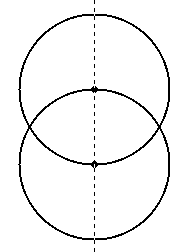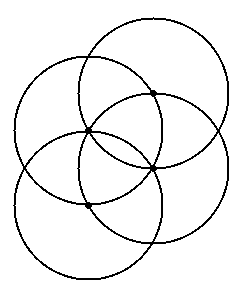Step 2: Vesica Piscis Step 3: Tripod of Life Step 4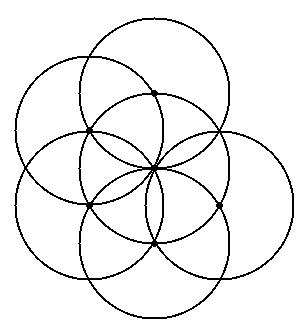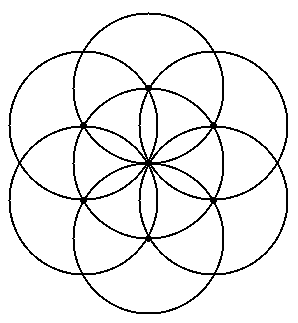Step 5 Step 6 Step 7: The Seed of Life Figure 2

3.- Egg of Life

The six outer circles in the Seed of Life intersect at six new points. These are the centers of the next iteration of circles. The final result is called the Egg of Life.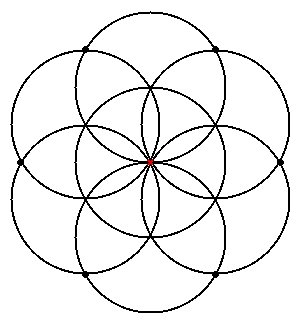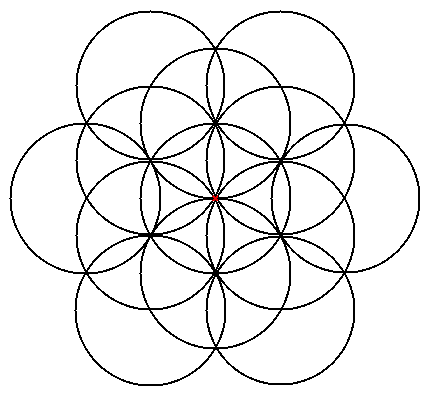(a) Outer intersecting points in the Seed of Life (b) The Egg of Life Figure 3

In this article we describe two possible three dimensional structures of the Egg of Life, with the inner grid that supports them. The final result is shown in Figure 4.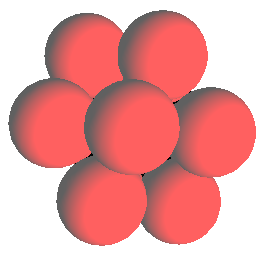(a) The Egg of Life as the vertices of a Vector Equilibrium or a Cuboctahedron with a central sphere. (b) The Egg of Life as the vertices of two intersecting tetrahedrons forming a Star Tetrahedron or a Cube. Figure 4

4.- Flower of Life

Starting from the Egg of life, another iteration of drawing circles centered at the outer intersecting points produces the central pattern in the Flower of Life. This pattern contains 19 full circles (Figure 5a). Then it is completed drawing half circles centered at the intersecting points of the external circles and at the tangent points with the outer enclosing circle. The "petals" of the outer "flowers" are also completed, and a second enclosing circle is drawn. The final result contains 19 circles each one with a "flower" within (Figure 5b).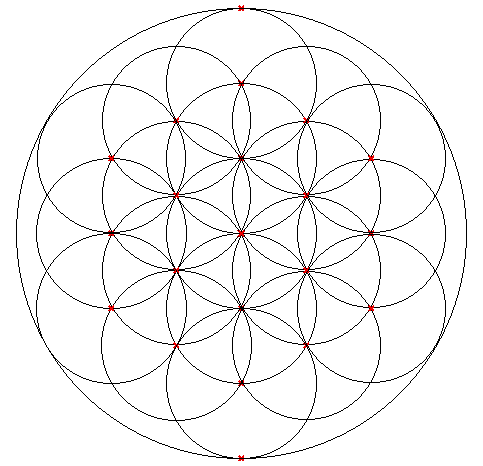(a) A next iteration starting from the Egg of Life produces the central pattern in the Flower of Life. (b) The Flower of Life Figure 5

5.- Metatron's Cube

The next two symbols are obtained by completing the outer circles in the Flower of Life. The first iteration produces what we hold is the 2D pattern of Metatron's Cube. If you give volume to the circles you end up with a 3D cube made of 4x4x4=64 spheres, the three dimensional Metatron's Cube. See this article for a detailed derivation (Figure 6).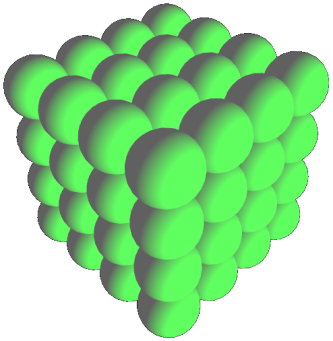(a) Two dimensional Metatron's Cube (b) Two dimensional Metatron's Cube Figure 6

6.- Fruit of Life

Completing the pattern in Figure 6a with another iteration of external circles produces a pattern which is known elsewhere as Metatron's Cube. This pattern contains three lines of five tangent circles known as the Fruit of Life (Figure 7).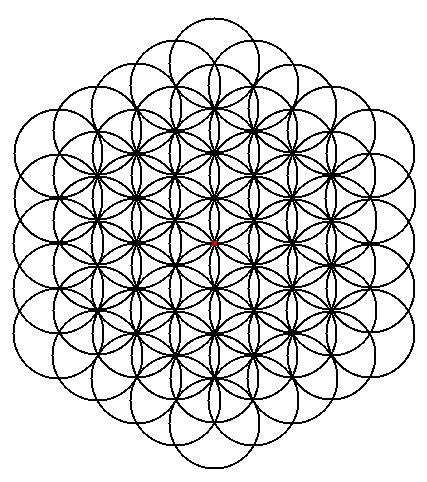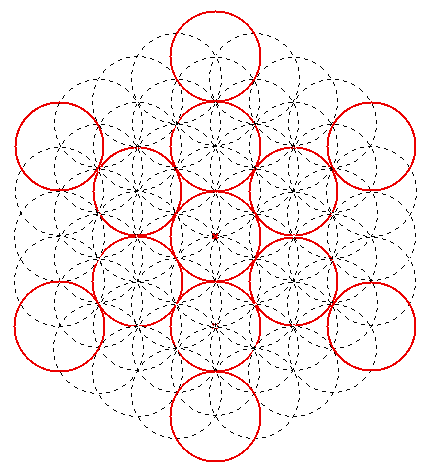(a) Final completion of the Flower of Life (b) The Fruit of Life Figure 7

In this article we describe two possible three-dimensional structures for the Fruit of Life (Figure 8). Both of them involve completing the pattern in Figure 7b with the six remaining tangent circles.(a) The Fruit of Life as the vertices of a big Vector Equilibrium, a fractal growth of Figure 4a doubling the edge size. (b) The Fruit of Life as the vertices of a cube made of 3x3x3 spheres, a fractal growth of Figure 4b doubling the edge size. Figure 8

The interested reader can take a look at this reference for further details on the conventional presentation of the Flower of Life.